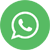The resultant model is a polynomial regression equation if the best fit line is a curve rather than a straight line

The R code for polynomial regression is a variant of the Linear regression Where the inputs are the polynomial function. For eg, if there is only one input X ten the function could be like this.

Im (y~X+l(x^2) +l(X^3))# this is for the third order polynomial

# Stepwise Regression

Stepwise Regression technique is processs where the choice of the input variable (inclusion or deletion of variables) by an automated process taking into considerthe t-statistic value of coefficients

The R code for the same is detailed below

start <-lm(Y~1)# model with no inputs end <-lm(Y~.)# model using all the inputs

#for forward selection step(start, scope=list(lower=start, upper=end),direction="forward")

#for backward elimination step(end, direction="backward") #for backward elimination the model with no inputs will not be needed

#for stepwise regression step(start,scope=list(upper=end), direction=both) The choice of the model (inputs) will be based on the least AIC value. Generally the last option in the output.

Ridge Regression

Ridge regression is variant of the linear regression used to circumvent to some extent the problem of multicollinearity problem amongst the inputs. The ridge regression adds a small factor of bias to the input variables in order to undertone the multicollinearity. The output of the ridge regression will create multiple set of coefficients for differnt 'tuning parameter'

lm.ridge(y~.,lambda =Seq(a,b,c))#a=start value; b=end value;c=increment step.lambda is ridge constant

Lasso Regression

Lasso stands of least absolute shrinkage & Selection operator. This technique performs both input selection and regularization for improved prediction accuracy. Lasso can be used for linear modelling and various generalised linear models.

The function for lasso in R is lars()

Elastic Net Regression

Elastic Net regression is also Lasso & Ridge regression as it tries to addres the inherent weaknesses or limitation of the Lasso Regression and Ridge regression.,

The function in r for Elastic Net regression is glmnet()